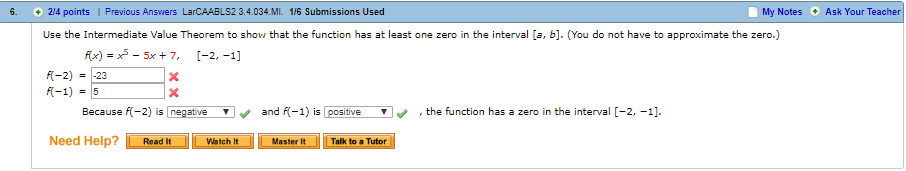6. 2/4 points | Previous Answers LarCAABLS2 3.4.034.MI 1/6 Submissions UsedMy Notes Ask Your TeacherUse the Intermediate Value Theorem to show that the function has at least one zero in the interval [a, b]. (You do not have to approximate the zero.)x) - 5x 7, I-2, -1]k-2) ,-23Because K-2) isnegativeand-1) is positive▼、, the function has a zero in the interval [-2.-11.Need Help? aWatchMatTal to TuterRead ItMaster It

Question

6,help_outlineImage Transcriptionclose6. 2/4 points | Previous Answers LarCAABLS2 3.4.034.MI 1/6 Submissions Used My Notes Ask Your Teacher Use the Intermediate Value Theorem to show that the function has at least one zero in the interval [a, b]. (You do not have to approximate the zero.) x) - 5x 7, I-2, -1] k-2) ,-23 Because K-2) is negative and -1) is positive ▼、, the function has a zero in the interval [-2.-11. Need Help? aWatchMatTal to Tuter Read It Master It fullscreen
Step 1

To show the existence of a solution to the given equation f(x)=0 over the given interval

Step 2

The problem is concerned with applying the intermediate value theorem: If f(x) is a continuous function on an interval [a,b] and if f(a) and f(b) have opposite signs,  then there exists a point c in [a,b] such that f(c)=0. (See the graphical interpretation)

Step 3

Apply the intermediate value theorem to our function f(x)=x^5-5x+7 over the interval [-2.-1]. f(x) ...

Want to see the full answer?

See Solution

Want to see this answer and more?

Our solutions are written by experts, many with advanced degrees, and available 24/7

See Solution
Tagged in

Algebra